# Simple circuit provides high-side current sensing

## Analog Devices OP90

,

In power supplies or battery chargers, you often need information about the current flowing in the high-side rail. Figure 1 shows a common circuit for obtaining this information.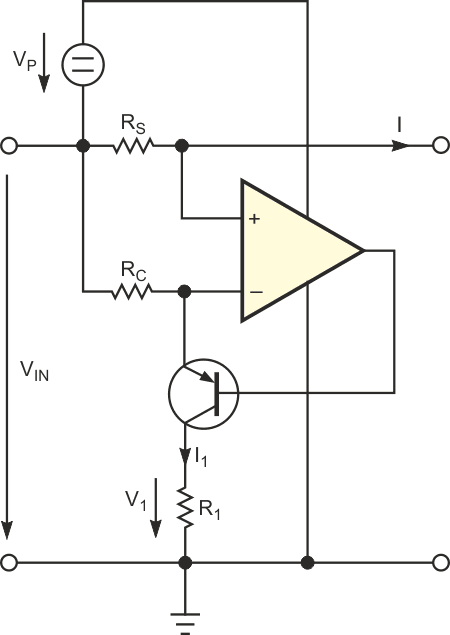Figure 1. This method for measuring high-side supply current requires an auxiliary transistor.

The circuit provides a ground-referenced voltage proportional to the current flowing through the high-side sense resistor, RS. The circuit needs an additional high-side supply, VP. If you use a low-voltage op amp, such as an OP90, VP = 1.5 V is adequate, so you can derive this supply from a series-transistor voltage drop in many applications. The op amp keeps the voltage drop in RS and the conversion resistor, RC, equal, so the current through R1 is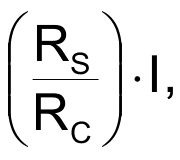and the voltage across R1 is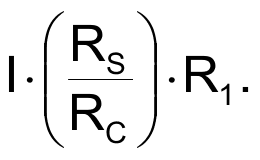Figure 2 shows a circuit that needs no auxiliary transistor. The output stage of the op amp, in a sense, replaces the transistor. The current through RC is the same as that in Figure 1. Now, this current flows through the negative-supply pin of the op amp and serves as the current source for R1 as before. The circuit uses a low-power OP90 op amp, which draws a low supply current. The idea is that the supply current contributes a negligible offset to I1. Measurements show that the positive-supply current, IP, rises with current I1. This situation leads to a higher current I1 because of the current IP. Figure 3 shows the result. The dots in Figure 3 indicate the measured values of V1, and the straight line shows the value if IP were zero.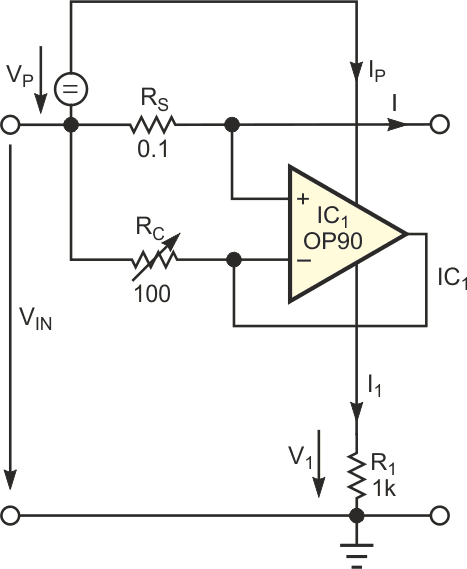Figure 2. This circuit uses the negative-supply current of the op amp to measure the high-side supply current.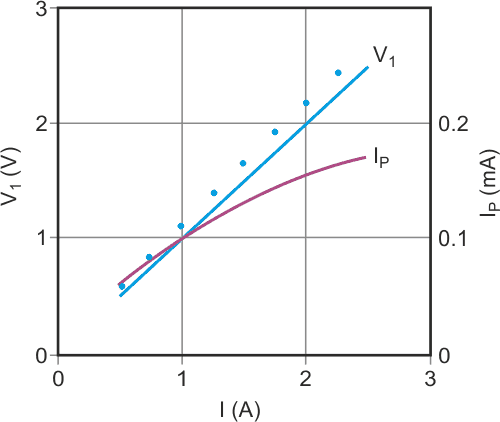Figure 3. Because of the positive-supply current, the measured values (dots) do not agree well with the theoretical values (straight line).

Fortunately, the supply, IP, is nearly proportional to the op amp's output current. So the value of RC in Figure 2 is such to yield the right value at the maximum current of 2.5 A. This simple, one-point adjustment corrects the gain error. Figure 4 shows the result after this gain compensation. Now, the error between the measured V1 and the desired value is within 5%, which is tolerable for many applications. Measurements show that the accuracy holds for input voltages of 5 to 25 V and an additional supply voltage of 2 to 15 .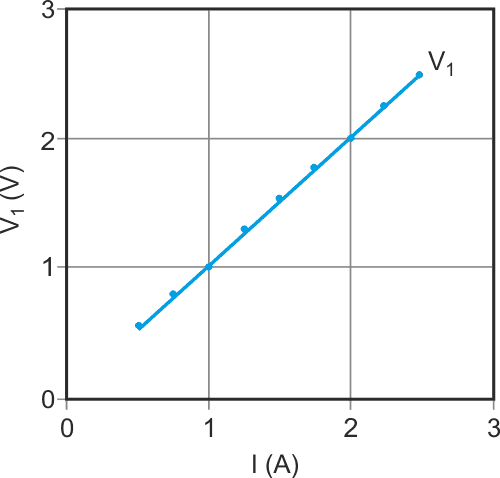Figure 4. Gain compensation by adjusting RC improves the accuracy of the measurement.

EDN